# three.js 制作机房（上）

By | 2020年11月21日

three.js使用的人太少了，一个博文就几百个人看，之前发js基础哪怕是d3都会有几千的阅读量，看看以后考虑说一说d3了，哈哈。吐槽完毕回归正题。前几天郭先生看到网上有人开发了3D机房，正愁博客没什么写的，于是昨天熬夜也做了一个，今天就把大体的流程告诉萌新们，先说说主要功能模块。

1. 墙体、地面、窗户以及门的实现（双击门禁门可开关）。
2. 机柜实现（机柜门的开关、机箱的推拉以及开关推拉的条件）。
3. 机箱存储占用比率（用颜色表示占用率，并附颜色谱图）。
4. 监控摄像视角（包括监控摄像机的模型导入，和四视角监控）。
5. 红外防控报警。
6. 强弱电线的铺设。
7. 以及风向。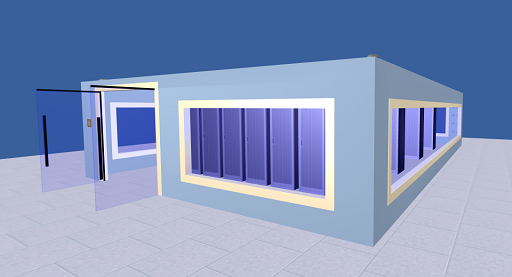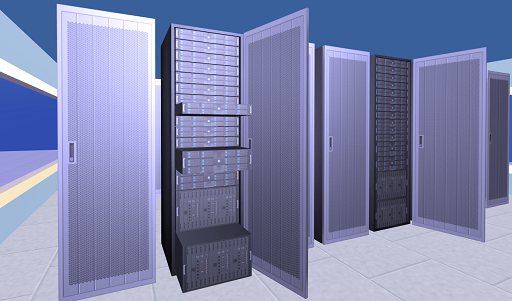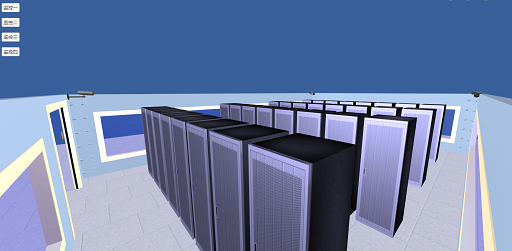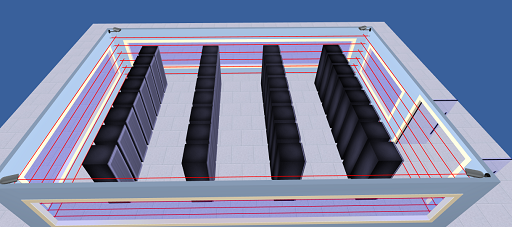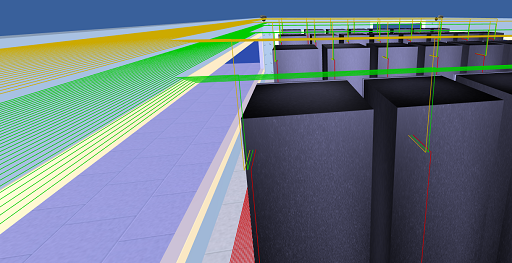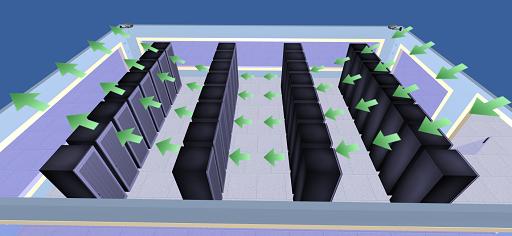## 1. 墙体、地面、窗户以及门的实现

### 1. 墙的实现

```var wallArr = [
{s: [1, 20, 61], p: [45, 10, 0], dir: 'z', hasDoor: true, ds: [1, 18, 18], dp: [45, 9, 15], hasWindow: true, ws: [1, 10, 24], wp: [45, 10, -13]},
{s: [1, 20, 61], p: [-45, 10, 0], dir: 'z', hasDoor: false, hasWindow: true, ws: [1.2, 10, 50], wp: [-45, 10, 0]},
// {s: [46, 20, 1], p: [22.5, 10, 30], dir: 'x', hasDoor: false, hasWindow: true, ws: [30, 10, 1], wp: [22.5, 10, 30]},
// {s: [46, 20, 1], p: [-22.5, 10, 30], dir: 'x', hasDoor: false, hasWindow: true, ws: [30, 10, 1], wp: [-22.5, 10, 30]},
// {s: [46, 20, 1], p: [22.5, 10, -30], dir: 'x', hasDoor: false, hasWindow: true, ws: [30, 10, 1], wp: [22.5, 10, -30]},
// {s: [46, 20, 1], p: [-22.5, 10, -30], dir: 'x', hasDoor: false, hasWindow: true, ws: [30, 10, 1], wp: [-22.5, 10, -30]},
{s: [91, 20, 1], p: [0, 10, 30], dir: 'x', hasDoor: false, hasWindow: true, ws: [80, 10, 1], wp: [0, 10, 30]},
{s: [91, 20, 1], p: [0, 10, -30], dir: 'x', hasDoor: false, hasWindow: true, ws: [80, 10, 1], wp: [0, 10, -30]}
];```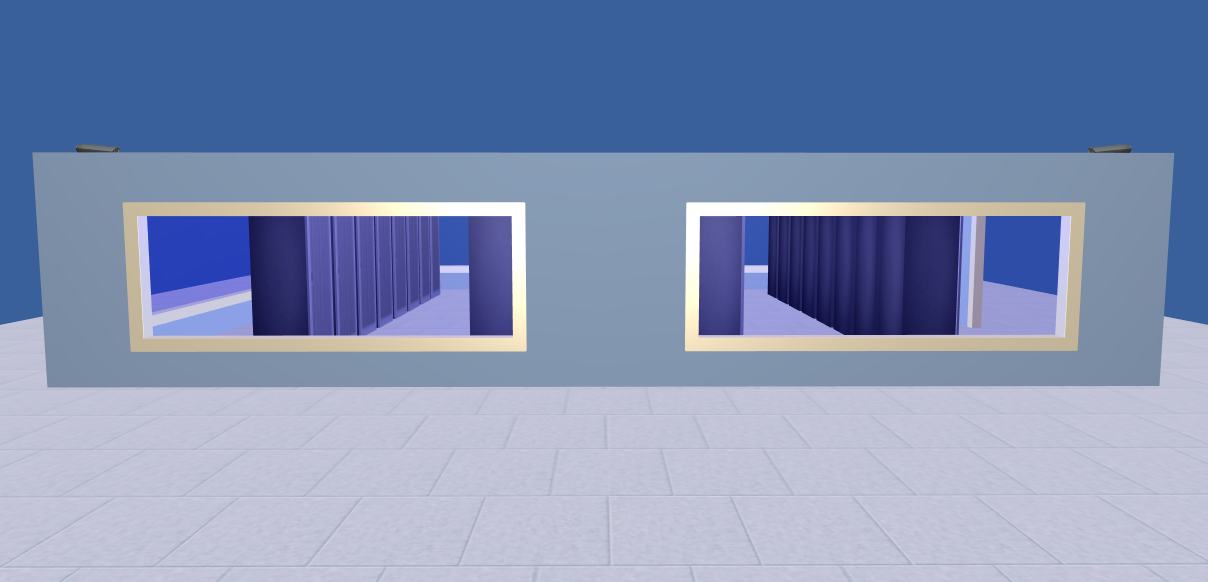ok测试没有问题。

### 2. 门的实现

```doorGeom1.faceVertexUvs = [new THREE.Vector2(1,1), new THREE.Vector2(1,0), new THREE.Vector2(0,1)];
doorGeom1.faceVertexUvs = [new THREE.Vector2(1,0), new THREE.Vector2(0,0), new THREE.Vector2(0,1)];
doorGeom2.faceVertexUvs = [new THREE.Vector2(1,1), new THREE.Vector2(1,0), new THREE.Vector2(0,1)];
doorGeom2.faceVertexUvs = [new THREE.Vector2(1,0), new THREE.Vector2(0,0), new THREE.Vector2(0,1)];```

### 3. 地面的实现

```floorT.wrapS = floorT.wrapT = THREE.RepeatWrapping;
floorT.repeat.x = floorT.repeat.y = 12;```

## 2. 机柜的实现

### 2. 服务器的实现

```for(let i=2; i) {
serGeom.faceVertexUvs[i] = [new THREE.Vector2(), new THREE.Vector2(), new THREE.Vector2()];
}```

### 3.服务器和柜门的点击事件

```let intersectFrameDoor = raycaster.intersectObjects(motorGroup.children, true);
let tempArr = intersectFrameDoor.object.name.split('-');//得到[机柜门/服务器名字,机柜编号,服务器编号[
if(tempArr == 'mdoor') {
if(!this.motorServerFlag[tempArr]) {
if(this.motorDoorFlag[tempArr]) {
this.doAnimate(Math.PI * 3 / 5, 0, 500, intersectFrameDoor.object.parent, ['rotation', 'y'])
this.motorDoorFlag[tempArr] = false;
} else {
this.doAnimate(0, Math.PI * 3 / 5, 500, intersectFrameDoor.object.parent, ['rotation', 'y'])
this.motorDoorFlag[tempArr] = true;
}
}
} else if(tempArr == 'mserver') {
if(this.motorDoorFlag[tempArr]) {
let posx = intersectFrameDoor.object.position.x;
if(posx == 0) {
this.doAnimate(0, 2, 500, intersectFrameDoor.object, ['position', 'x']);
this.motorServerFlag[tempArr] += 1;
} else {
this.doAnimate(2, 0, 500, intersectFrameDoor.object, ['position', 'x']);
this.motorServerFlag[tempArr] -= 1;
}
}
}```

### 4. 封装动画

1. 关键帧动画
2. Tween动画
3. 自制动画

```doAnimate(s, e, t, o, a) { //开始的属性值、结束的属性值 时间 对象 属性
let temp = s;
let step = t / 20;
let stepLen = (e - s) / step;
let animationObj = setInterval(() => {
temp += stepLen;
if(stepLen > 0 && temp >= e) {
o[a][a] = e;
clearInterval(animationObj);
} else if(stepLen  e) {
o[a][a] = e;
clearInterval(animationObj);
} else {
o[a][a] = temp;
}
}, 20)
}```

?